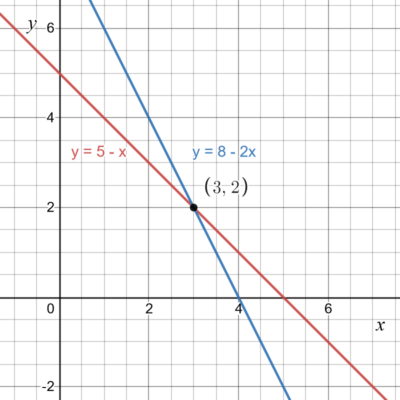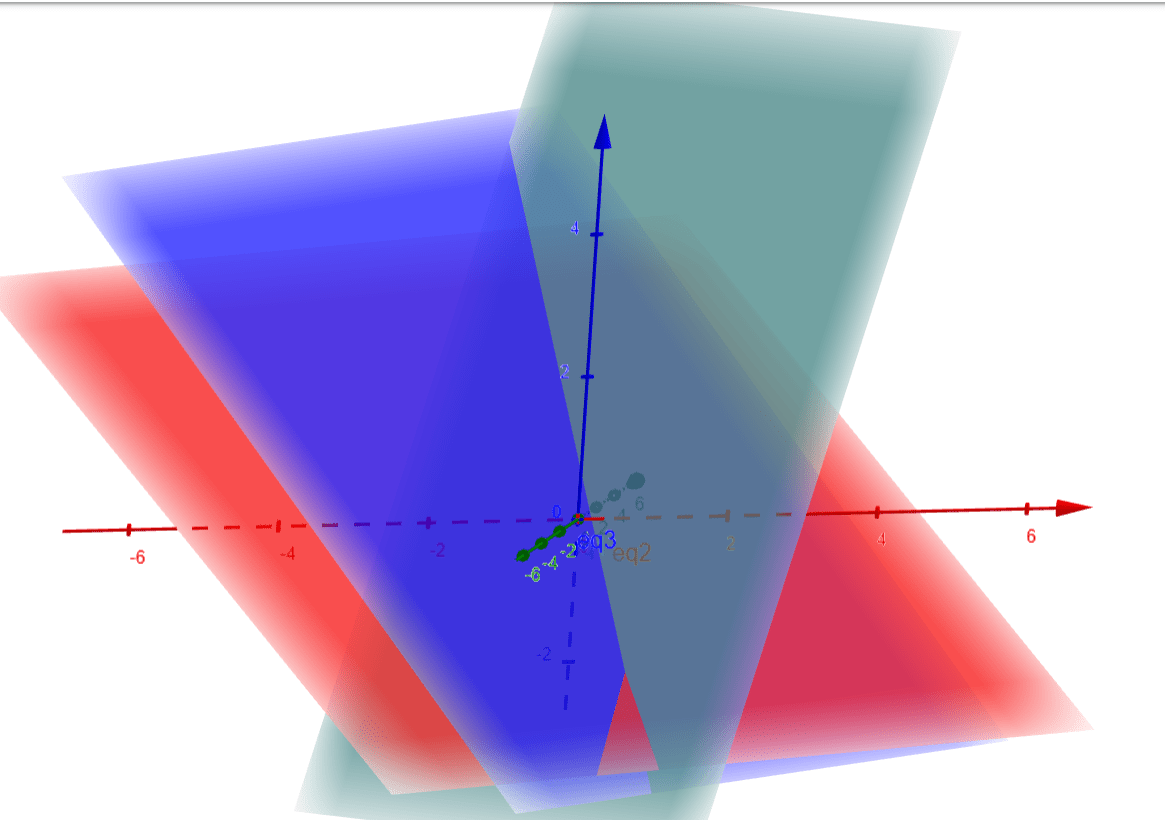Introduction to System of Linear Equations

What is a system of linear equations? A system of linear equations is a set of equations with more than one variable. There can be either no solution, one unique solution or multiple solutions for the system. For now, we will assume that all examples will have strictly one unique solution. An example,$x + y = 5$$2x + y = 8$

is a system of 2 equations with variables of x and y. When solving this, you must consider all of
the equations involved and find a solution that satisfies all of the equations. In S2, these equations can be represented with AbstractRealScalarFunction, which accepts a parameter for the number of variables that are in the equation.
				
%use s2
//define function x + y = 5
val f1:RealScalarFunction = object : AbstractRealScalarFunction(2) {
//setting the value as 2 for the 2 variables
override fun evaluate(x: Vector): Double {
val x:Double = x.get(1)
val y:Double = x.get(2)
//if the values are correct, it returns 0
return x + y - 5
}}




Graphing Method

A way to visualize this is with the use of a graph. In graph, these equations would be represented as$y = 5-x$$y = 8-2x$

Which can be also displayed as,From the above graphs, you can see that the x and y values where both equations will be fulfilled is at the point of intersection, which is x = 3, y = 2. To prove that the solution is valid, you can substitute x = 3 and y = 2,$2 = 5-3$$2 = 8-3*2$

Hence, the system of equations is valid when x = 3, y = 2.

System of 3 or more variables

There can be more than just 2 variables in a system of equation. For example,$3x+2y-z=1$$2x-2y+4z=-2$$-x+1/2 y-z=0$

is a system of 3 equations with 3 variables, x, y and z. In a system with 3 variables, you can use a 3D graph to visualize the 3 equations using x, y and z as the vertices.This would be a visualisation of the system, with the point of intersection being the solution. In this case,$x=1$$y=-2$$z=-2$

is the solution to the system. This is because the point of intersection is the only point where the values of x, y and z holds true for all the equations in the system. In essence, no matter the number of variables, you are able to determine if a set of numbers is the solution if all equations in a system of equations holds true with the set of numbers.

Alternate solutions

Generally, there are three ways a system of linear equations can behave.

1. The system has a single unique solution. (All graphs intersects at one unique point)
2.
The system has infinitely many solutions. (All graphs of the equations are on the same line)
3. The system has no solution. (All graphs do not intersect at any point)

We have thus far been working with the assumption that the system follows the first behavior mentioned for all equations, but there are other types of systems to take note. For the below examples, we will use only 2 variables, x and y, for ease of plotting graphs and to better showcase the relationships between the lines.

Infinitely many solutions

A system which has infinitely many solutions is a system where all the equations are valid at every possible value. Graphically, the equations will be on the same line. For example,$y=5-x$$2y=10-2x$

is a system where the both equations share the same line. The graph will look like this.With this system, there will be infinite solutions as there is a point of intersection for each and every value of x, hence every value of x can be the solution.

No solutions

A system which has no solutions is a system in which there are no values for the variables where the equations will be valid. Graphically, there will be no point of intersection where all the graphs pass through. For example,$y=2x+3$$y=2x+5$$y=-x+1$

is a system which will have no solutions as there is no point at which all 3 equations intersect.With this system, there is no solutions as there is no point on the graph where the x and y values are constant for all equations in the system.